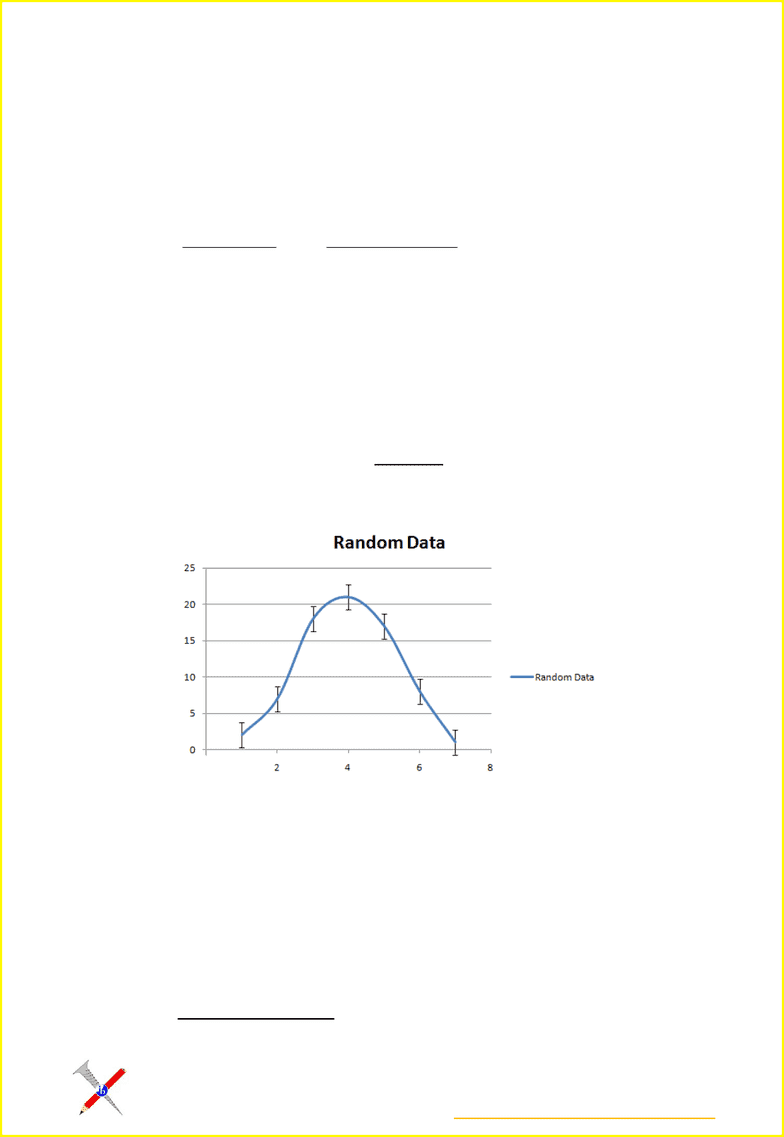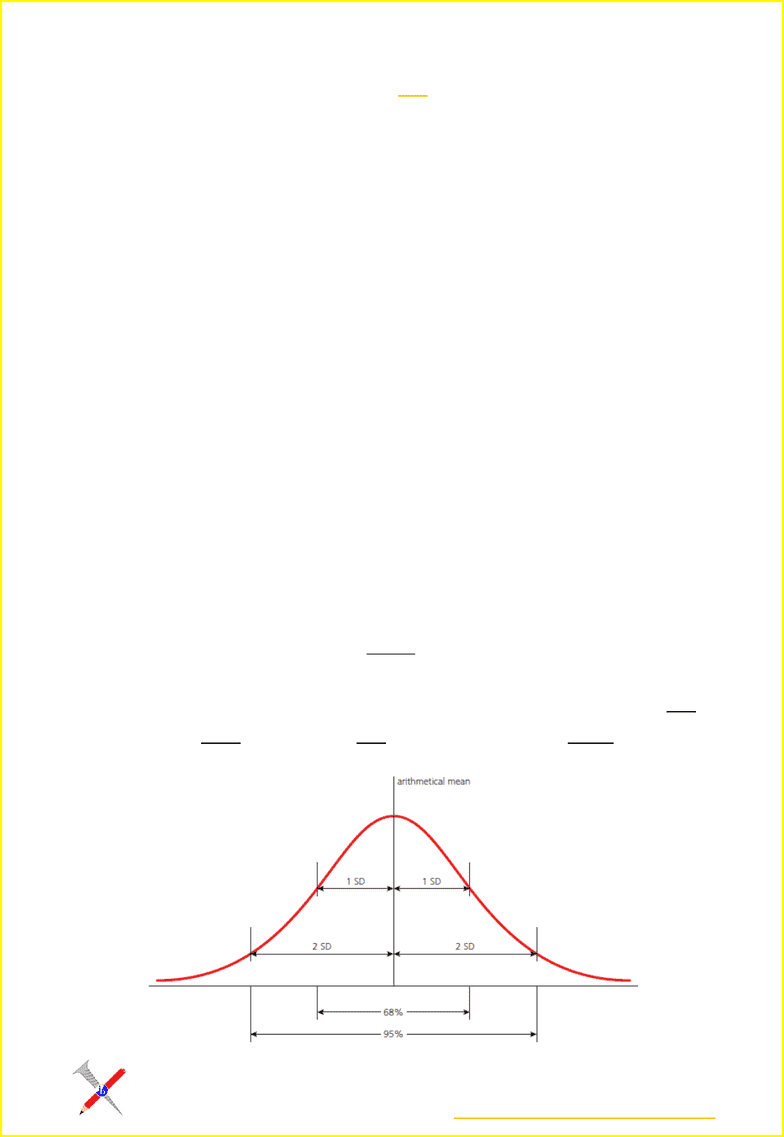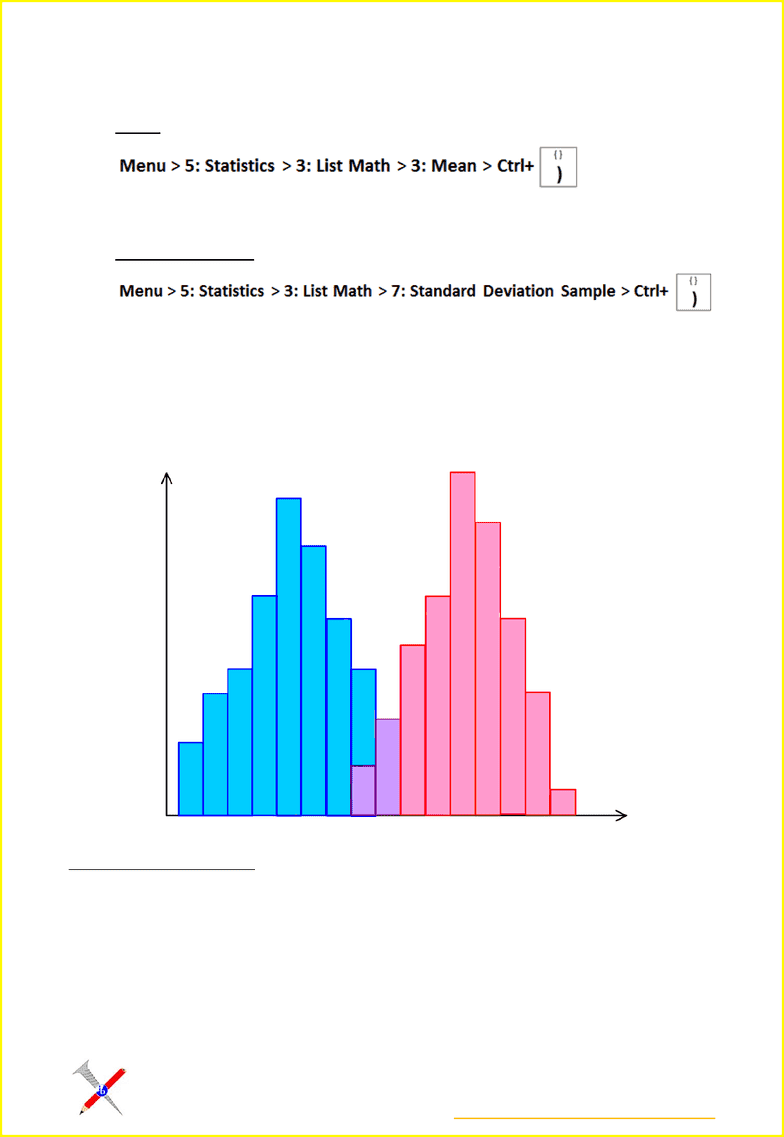Class Notes (1,100,000)
CA (620,000)
McMaster (50,000)
BIOLOGY (2,000)
Lecture 1

# BIOLOGY 1A03 Lecture Notes - Lecture 1: Error Bar, Standard Deviation, Null Hypothesis

Department
Biology
Course Code
BIOLOGY 1A03
Professor
Lovaye Kajiura
Lecture
1

This preview shows pages 1-3. to view the full 10 pages of the document.http://ibscrewed4biology.blogspot.com/
1.1 Statistical Analysis
1.1.1 - State that error bars are a graphical representation of the variability of data
Error bars are a graphical representation of the variability of data. Error bars can be used to
show either the range of data or the standard deviation on a graph. The value extending
above and below one bar will be the same.
When we collect data, it will always variation data because biological systems are subject to
a genetic program and environmental variation.
Time is sometimes too limited to be able to repeat experiments enough time to confirm an
accurate result. Instead, we display data, we show the degree of variability in the readings.
When we are doing this on a graph, we use error bars. Each error bar represents the range
of readings obtained for that value, or the standard deviation.
1.1.2 - Calculate the mean and standard deviation of a set of values
Mean
The mean is a measure of the central tendency of the data. If the distribution is skewed, the
mean may not in fact be the middle value. The median or mode may be more appropriate.
The mean is an average of data points. To calculate a mean value, all the values are
summed, and the total divided by the number of values.

Only pages 1-3 are available for preview. Some parts have been intentionally blurred.http://ibscrewed4biology.blogspot.com/
= arithmetic mean
= sum of all measurements
= the total number of measurements
Standard Deviation
The standard deviation is a measure of how the individual observations of a data set are
dispersed or spread around the mean. Standard deviation is determined by a mathematical
formula which is programmed into your calculator.
The standard deviation of the mean tells us how spread out the readings are. A small
standard deviation indicates that the data is clustered closely around the mean value, whilst
a large standard deviation indicates a wider spread around the mean
To calculate this by hand:
1. 
2. 
3. 
4. 
5. 
Once obtained, the value may be applied to the normal distribution curve. Note that 68% of
data occurs within ±1 SD, and more than 95% of the data occurs within ±2 SDs.

Only pages 1-3 are available for preview. Some parts have been intentionally blurred.http://ibscrewed4biology.blogspot.com/
To calculate using the Graphics Calculator (TI-nspire©):
Mean:
Then simply enter the numbers, separated by commas, and press Enter
Standard Deviation:
Then enter the numbers, separated by commas, and press Enter
Mean in Experimental situations
Mean, Median and Mode -
Mode is the most frequent in a set of values
Median is the middle value when in a set of values arranged in ascending order
Mean is the sum of all the values divided by the number of values
Range is the spread of the data, measured by the difference between the highest and
lowest values. This is especially affected by any outliers.